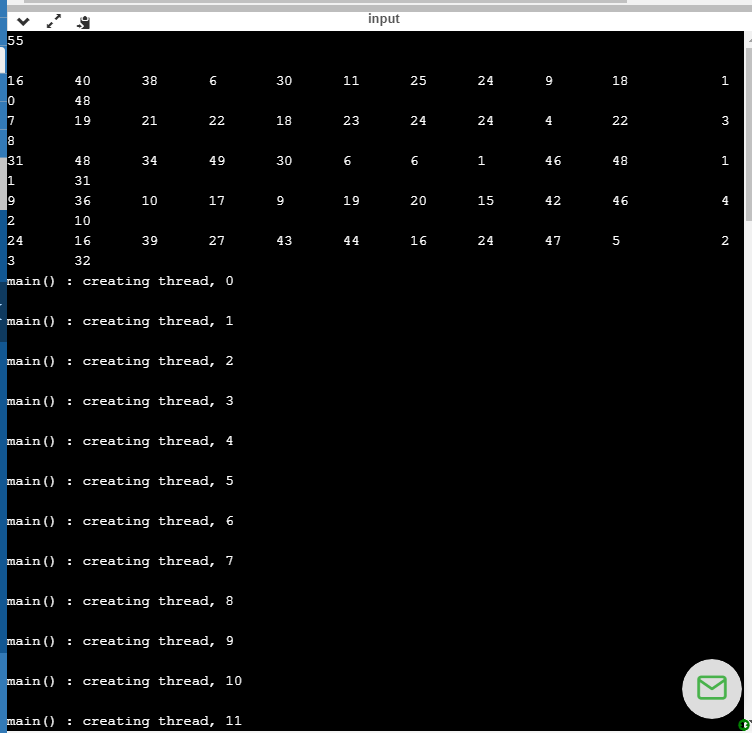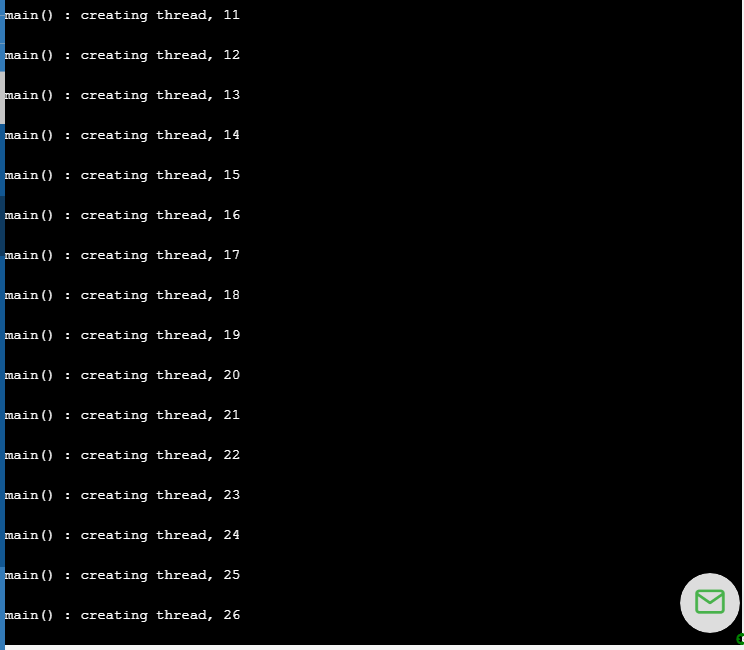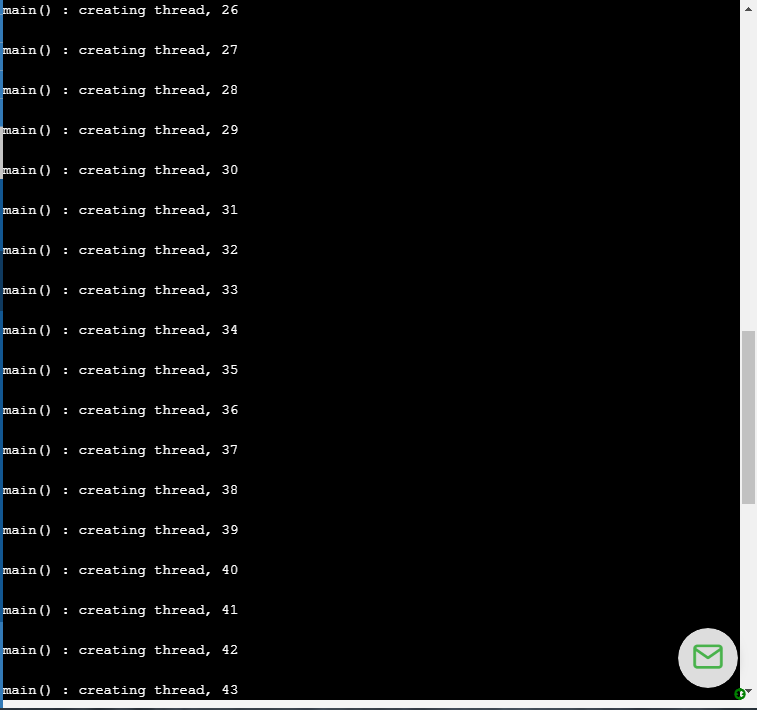In: Other

# Write a C or C++ program A6p2.c(pp) that accepts one command line argument which is an integer n between 2 and 4 inclusi...

Write a C or C++ program A6p2.c(pp) that accepts one command line argument which is an integer n between 2 and 4 inclusive. Generate 60 random integers between 1 and 49 inclusive and store them in a 5 by 12 two dimensional integer array (e.g.,int a;). Use pthread to create n threads to square all 60 array elements. You should divide this update task among the n threads as evenly as possible. Print the array both before and after the update separately as 5 by 12 matrices.

## Solutions

##### Expert Solution

#include <iostream>
#include <cstdlib>

using namespace std;

#define COLS 12
#define ROWS 5

int chunkSize;
int n=0;
int arr[ROWS][COLS] ;
long tid;

int start = tid * chunkSize;
int end=start+chunkSize-1;

for(int i=0; i<ROWS; i++){

for(int j=0;j<COLS;j++){

int position = i*COLS + j;

if(position>=start&&position<=end)
{
arr[i][j]=arr[i][j]*arr[i][j];
}
}

}

}

int main () {

cin>>n;
int rc;
int i,j,k;

//fill array wil data
for(i=0;i<ROWS;i++)
{
for(j=0;j<COLS;j++)
{
arr[i][j]=(rand() % 49) + 1;
}
}

//Printing Random Array
for(i=0;i<ROWS;i++)
{
cout<<"\n";
for(j=0;j<COLS;j++)
{
cout<<arr[i][j]<<"\t";
}
}

chunkSize = (60 + n - 1) / n; // divide by threads rounded up.

for( i = 0; i < n; i++ ) {
cout << "\nmain() : creating thread, " << i << endl;

if (rc) {
cout << "Error:unable to create thread," << rc << endl;
exit(-1);
}
}
//Printing Resulter Array
for(i=0;i<ROWS;i++)
{
cout<<"\n";
for(j=0;j<COLS;j++)
{
cout<<arr[i][j]<<"\t";
}
}
}## Related Solutions

##### Write a java code that: 1) Takes as an argument an integer number, say N 2)...
Write a java code that: 1) Takes as an argument an integer number, say N 2) Creates an array of size N 3) Populates the array with random numbers between 0 and 10 * N. This is, the values of the elements of the array should be random numbers between 0 and 10 * N. 4) Finally, the code outputs the index of the smallest element and the index of the largest element in the array
##### Initialize and Print an Array Write a program that accepts two integer values, called "arraySize" and...
Initialize and Print an Array Write a program that accepts two integer values, called "arraySize" and "multiplier", as user input. Create an array of integers with arraySize elements. Set each array element to the value i*multiplier, where i is the element's index. Next create two functions, called PrintForward() and PrintBackward(), that each accept two parameters: (a) the array to print, (b) the size of the array. The PrintForward() function should print each integer in the array, beginning with index 0....
##### C++ Write a program that takes a string and integer as input, and outputs a sentence...
C++ Write a program that takes a string and integer as input, and outputs a sentence using those items as below. The program repeats until the input string is "quit". If the input is: apples 5 shoes 2 quit 0 the output is: Eating 5 apples a day keeps your doctor away. Eating 2 shoes a day keeps your doctor away.
##### Write an interactive Java class which accepts an input argument when the application is executed from...
Write an interactive Java class which accepts an input argument when the application is executed from the command-line. Accept input from the user and compare the value entered to the command-line argument value. If the strings do not equal, display "INVALID VALUE! TRY AGAIN!", otherwise display "PERMISSION GRANTED!" and exit the program.
##### Program must be in C++! Write a program which: Write a program which uses the following...
Program must be in C++! Write a program which: Write a program which uses the following arrays: empID: An array of 7 integers to hold employee identification numbers. The array should be initialized with the following values: 1, 2, 3, 4, 5, 6, 7. Hours: an array of seven integers to hold the number of hours worked by each employee. payRate: an array of seven doubles to hold each employee’s hourly pay rate. Wages: an array of seven doubles to...
##### JAVA Language: Write a program that prompts the user to enter a positive integer n (0...
JAVA Language: Write a program that prompts the user to enter a positive integer n (0 up to 232 -1). You must write a function that takes as input n and returns a string s representing the number n in binary. For this assignment, you must use the method of successive division by 2 to convert the number to binary. Your main program must print out s. Example: If the user enters the number 66, your program must print out...
##### 1. Write a program in C++ that takes as inputs a positiveinteger n and a...
1. Write a program in C++ that takes as inputs a positive integer n and a positive double a. The function should compute the geometric sum with base a up to the powern and stores the result as a protected variable. That is, the sum is: 1 + ? + ? ^2 + ? ^3 + ? ^4 + ⋯ + ? ^?2.  Write a program in C++ that takes as input a positive integer n and computes the following productsum...
##### (IN C) Program Question 2: Write a program that solves for c in the Pythagorean Theorem:...
(IN C) Program Question 2: Write a program that solves for c in the Pythagorean Theorem: a2 + b2 = c2 The user will enter values for a and b, and you will calculate c. All of this code will go in just one source file.
##### USING C# 1. Write a program that takes outputs a string, an integer and a floating-point...
USING C# 1. Write a program that takes outputs a string, an integer and a floating-point number separated by commas. Sample output: Bob Marley, 20, 5.2 2. 2. Write a program that asks the user for a string of letters of any size (no spaces), and finally a special character (values 33 to 47 in the Ascii table, or ‘!’ to ‘/’). Generate a random number of any size, integer or floating point, and combine those three pieces of information...
##### [C] Write a program that takes in a positive integer as input, and outputs a string of 1's and 0's representing the integer in binary. For an integer x, the algorithm is:
6.19 LAB: Convert to binary - functionsWrite a program that takes in a positive integer as input, and outputs a string of 1's and 0's representing the integer in binary. For an integer x, the algorithm is:As long as x is greater than 0    Output x % 2 (remainder is either 0 or 1)    x = x / 2Note: The above algorithm outputs the 0's and 1's in reverse order. You will need to write a second function to reverse the string.Ex: If the input is:6the output is:110Your program must define and call the following two functions. The IntegerToReverseBinary function...